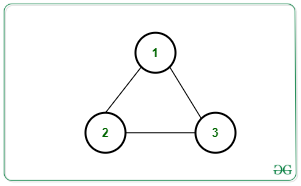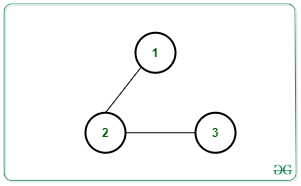Minimum number of edges required to be removed from an Undirected Graph to make it acyclic

• Difficulty Level : Medium
• Last Updated : 21 Jun, 2021

Given an undirected graph consisting of N nodes containing values from the range [1, N] and M edges in a matrix Edges[][], the task is to determine the minimum number of edges required to be removed such that the resulting graph does not contain any cycle.

Examples:

Input: N = 3, M = 3, edges[][] = [[1, 2], [2, 3], [3, 1]]Output: 1
Explanation:
Removing any one of the edges will make the graph acyclic. Therefore, at least one edge needs to be removed.

Input: N = 3, M = 2, edges[][] = [[1, 2], [2, 3]]Output: 0
Explanation: Graph is already acyclic. Therefore, no edge removal is required.

Naive Approach: The simplest approach is to try deleting all possible combination of sequence of edges from the given graph one by one and for each combination, count the number of removals required to make the graph acyclic. Finally, among these combinations, choose the one which deletes the minimum number of edges to obtain an acyclic graph.
Time Complexity: O(M!)
Auxiliary Space: O(N + M)

Efficient Approach: The above approach can be optimized based on the following observations:

1. A graph is acyclic when it is a Tree or a forest of trees(disconnected groups of trees).
2. A tree with C nodes will have (C – 1) edges.
3. If there are K connected components from C1 to CK, then minimum number of edges to be removed is equal to:

M – (C1 – 1) – (C2 – 1) … (Ck -1 )
=> M – (C1 + … + CK) + K
=> M – N + K

Follow the steps below to solve the problem:

1. Find the number of connected components from the given graph using DFS.
2. Considering the count of connected components to be K, then print M – N + K as the required minimum number of edges to be removed to make the resulting graph acyclic.

Below is the implementation of the above approach:

C++

 // C++ Program to implement// the above approach #include using namespace std; // Stores the adjacency listvector vec; // Stores if a vertex is// visited or notbool vis;int cc = 1; // Function to perform DFS Traversal// to count the number and size of// all connected componentsvoid dfs(int node){    // Mark the current node as visited    vis[node] = true;     // Traverse the adjacency list    // of the current node    for (auto x : vec[node]) {         // For every unvisited node        if (!vis[x]) {            cc++;             // Recursive DFS Call            dfs(x);        }    }} // Function to add undirected// edge in the graphvoid addEdge(int u, int v){    vec[u].push_back(v);    vec[v].push_back(u);} // Function to calculate minimum// number of edges to be removedvoid minEdgeRemoved(int N, int M,                    int Edges[]){     // Create Adjacency list    for (int i = 0; i < M; i++) {        addEdge(Edges[i],                Edges[i]);    }     memset(vis, false, sizeof(vis));    int k = 0;     // Iterate over all the nodes    for (int i = 1; i <= N; i++) {        if (!vis[i]) {            cc = 1;            dfs(i);            k++;        }    }     // Print the final count    cout << M - N + k << endl;} // Driver Codeint main(){    int N = 3, M = 2;     int Edges[] = { { 1, 2 }, { 2, 3 } };     minEdgeRemoved(N, M, Edges);}

Java

 // Java program to implement// the above approachimport java.util.*; class GFG{ // Stores the adjacency list@SuppressWarnings("unchecked")static Vector []vec = new Vector; // Stores if a vertex is// visited or notstatic boolean []vis = new boolean;static int cc = 1; // Function to perform DFS Traversal// to count the number and size of// all connected componentsstatic void dfs(int node){         // Mark the current node as visited    vis[node] = true;     // Traverse the adjacency list    // of the current node    for(int x : vec[node])    {                 // For every unvisited node        if (!vis[x])        {            cc++;             // Recursive DFS call            dfs(x);        }    }} // Function to add undirected// edge in the graphstatic void addEdge(int u, int v){    vec[u].add(v);    vec[v].add(u);} // Function to calculate minimum// number of edges to be removedstatic void minEdgeRemoved(int N, int M,                           int Edges[][]){         // Create Adjacency list    for(int i = 0; i < M; i++)    {        addEdge(Edges[i],                Edges[i]);    }     int k = 0;     // Iterate over all the nodes    for(int i = 1; i <= N; i++)    {        if (!vis[i])        {            cc = 1;            dfs(i);            k++;        }    }     // Print the final count    System.out.print(M - N + k + "\n");} // Driver Codepublic static void main(String[] args){    int N = 3, M = 2;     int Edges[][] = { { 1, 2 }, { 2, 3 } };         for(int i = 0; i < vec.length; i++)        vec[i] = new Vector();             minEdgeRemoved(N, M, Edges);}} // This code is contributed by 29AjayKumar

Python3

 # Python3 program to implement# the above approach # Stores the adjacency listvec = [[] for i in range(100001)] # Stores if a vertex is# visited or notvis = [False] * 100001cc = 1 # Function to perform DFS Traversal# to count the number and size of# all connected componentsdef dfs(node):         global cc         # Mark the current node as visited    vis[node] = True     # Traverse the adjacency list    # of the current node    for x in vec[node]:         # For every unvisited node        if (vis[x] == 0):            cc += 1             # Recursive DFS Call            dfs(x) # Function to add undirected# edge in the graphdef addEdge(u, v):         vec[u].append(v)    vec[v].append(u) # Function to calculate minimum# number of edges to be removeddef minEdgeRemoved(N, M, Edges):         global cc     # Create Adjacency list    for i in range(M):        addEdge(Edges[i], Edges[i])     # memset(vis, false, sizeof(vis))    k = 0     # Iterate over all the nodes    for i in range(1, N + 1):        if (not vis[i]):            cc = 1            dfs(i)            k += 1     # Print the final count    print(M - N + k) # Driver Codeif __name__ == '__main__':         N = 3    M = 2     Edges = [ [ 1, 2 ], [ 2, 3 ] ]     minEdgeRemoved(N, M, Edges) # This code is contributed by mohit kumar 29

C#

 // C# program to implement// the above approachusing System;using System.Collections.Generic; class GFG{ // Stores the adjacency liststatic List []vec = new List; // Stores if a vertex is// visited or notstatic bool []vis = new bool;static int cc = 1; // Function to perform DFS Traversal// to count the number and size of// all connected componentsstatic void dfs(int node){         // Mark the current node as visited    vis[node] = true;     // Traverse the adjacency list    // of the current node    foreach(int x in vec[node])    {                 // For every unvisited node        if (!vis[x])        {            cc++;             // Recursive DFS call            dfs(x);        }    }} // Function to add undirected// edge in the graphstatic void addEdge(int u, int v){    vec[u].Add(v);    vec[v].Add(u);} // Function to calculate minimum// number of edges to be removedstatic void minEdgeRemoved(int N, int M,                           int [,]Edges){         // Create Adjacency list    for(int i = 0; i < M; i++)    {        addEdge(Edges[i, 0],                Edges[i, 1]);    }     int k = 0;     // Iterate over all the nodes    for(int i = 1; i <= N; i++)    {        if (!vis[i])        {            cc = 1;            dfs(i);            k++;        }    }     // Print the readonly count    Console.Write(M - N + k + "\n");} // Driver Codepublic static void Main(String[] args){    int N = 3, M = 2;     int [,]Edges = { { 1, 2 }, { 2, 3 } };         for(int i = 0; i < vec.Length; i++)        vec[i] = new List();             minEdgeRemoved(N, M, Edges);}} // This code is contributed by 29AjayKumar

Javascript


Output:
0

Time Complexity: O(N + M)
Auxiliary Space: O(N + M)

My Personal Notes arrow_drop_up